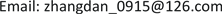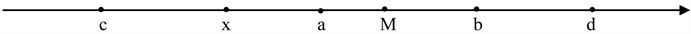﻿ 基于可变模糊集合理论的农业生态环境评估系统研究 Research on Agricultural Ecological Environment Assessment System Based on Variable Fuzzy Set Theory

Hans Journal of Agricultural Sciences
Vol.08 No.04(2018), Article ID:24445,9 pages
10.12677/HJAS.2018.84042

Research on Agricultural Ecological Environment Assessment System Based on Variable Fuzzy Set Theory

Dan Zhang1,2

1Research Institute of Water Resources and Hydropower in Liaoning Province, Shenyang Liaoning

2College of Water Conservancy, Shenyang Agricultural University, Shenyang LiaoningReceived: Mar. 30th, 2018; accepted: Apr. 16th, 2018; published: Apr. 23rd, 2018ABSTRACT

In order to alleviate water resources shortage and to play land resources’ advantages, our country implements “water saving and grain increasing action” in the four provinces of the northeast. But what’re the impacts on region’s soil, society, ecology and water environment to carry out a large range of groundwater as the main water source of the drip irrigation is a hot issue. In this paper, a comprehensive evaluation of water, soil, social and ecological environment in Jianping of Liaoning province was made based on variable fuzzy set theory. The results show that drip irrigation will reduce pesticide and fertilizer, and reduce soil and water environment pollution compared with pipe irrigation on agricultural ecological environment, level 1 lower than pipe irrigation. But drip irrigation needs to be covered with plastic film, and plastic recycling rate reached about 90 percent. In a long term, the remaining quantity will increase with the film content increasing, but the specific effects still need long-term observation.

Keywords:Water Saving and Grain Increasing, Variable Fuzzy Set, Agricultural Ecological Environment

1辽宁省水利水电科学研究院，辽宁 沈阳

2沈阳农业大学水利学院，辽宁 沈阳1. 引言

2. 可变模糊集合简介

$\left\{\begin{array}{l}{D}_{\underset{¨}{A}}\left(u\right)={\left(\frac{x-a}{M-a}\right)}^{\beta };\text{\hspace{0.17em}}x\in \left[a,M\right]\\ {D}_{\underset{¨}{A}}\left(u\right)=-{\left(\frac{x-a}{c-a}\right)}^{\beta };\text{\hspace{0.17em}}x\in \left[c,a\right]\end{array}$ (1)

$\left\{\begin{array}{l}{D}_{\underset{¨}{A}}\left(u\right)={\left(\frac{x-b}{M-b}\right)}^{\beta };\text{\hspace{0.17em}}x\in \left[M,b\right]\\ {D}_{\underset{¨}{A}}\left(u\right)=-{\left(\frac{x-b}{d-b}\right)}^{\beta };\text{\hspace{0.17em}}x\in \left[b,d\right]\end{array}$ (2)

${u}_{h}=\frac{1}{1+{\left\{\frac{\sum _{i=1}^{m}{\left[{w}_{i}\left(1-{\mu }_{h}\left({u}_{ij}\right)\right)\right]}^{p}}{\sum _{i=1}^{m}{\left({w}_{i}{\mu }_{h}\left({u}_{ij}\right)\right)}^{p}}\right\}}^{\alpha /p}}$ (3)

$\left\{\begin{array}{c}\alpha =1\\ p=1\end{array};\text{\hspace{0.17em}}\text{\hspace{0.17em}}\left\{\begin{array}{c}\alpha =1\\ p=2\end{array};\text{\hspace{0.17em}}\text{\hspace{0.17em}}\left\{\begin{array}{c}\alpha =2\\ p=2\end{array};\text{\hspace{0.17em}}\text{\hspace{0.17em}}\left\{\begin{array}{c}\alpha =2\\ p=2\end{array}$ .

${v}_{\underset{˜}{A}}\left(u\right)=\left({u}_{1},{u}_{2},\cdots ,{u}_{h}\right)$ (4)

${H}_{j}=\left(\begin{array}{cccc}1& 2& \cdots & c\end{array}\right)\cdot {v}_{\underset{˜}{A}}{\left(u\right)}_{h}^{\text{T}}$ (5)

3. 基于可变模糊集合的农业生态环境评估系统研究

3.1. 农业生态环境评估指标体系

1) 水与生物环境系统指标体系Figure 1. Position relation of the point x, M and [a,b], [c,d]Figure 2. Index system of agricultural ecological environmental assessment systemTable 1. Level variable range of agricultural ecological environment

2) 土壤环境系统指标体系

3) 社会环境系统指标体系

3.2. 农业生态环境评价指标等级划分

3.3. 指标权重的确定

$w=\alpha {w}_{1}+\left(1-\alpha \right){w}_{2}$ (6)

${w}_{1}$ ——评价指标的经验权重；

${w}_{2}$ ——评价指标的数学权重；

a——灵敏度系数， $0<\alpha <1$ 。一般情况下，a取值范围为0.5~0.7，本文选取中间值0.6作为组合权重的灵敏度系数。

3.4. 研究结果

3.4.1. 农业生态环境指标特征值Table 2. Index system classification of agricultural ecological environmentTable 3. Evaluation indexes of agricultural ecological environment of Changlong in 2015

3.4.2. 指标权重的确定

1) 数学权重的确定

$p=⌊\begin{array}{cccccc}0.4483& 0.28& 1& 1& 1& 1\\ 0& 0& 0& 0.7204& 0.7197& 0\\ 1& 1& 0.9684& 0& 0& 0\end{array}⌋$

2) 经验权重的确定

${\omega }_{2}=\left(0.2139,0.1500,0.1722,0.1389,0.1361,0.1889\right)$

3) 综合权重的确定

$\omega =\left(0.1940,0.1565,0.1699,0.1490,0.1473,0.1832\right)$

${\mu }_{\underset{_}{A}}^{0}\left({x}_{j}\right)=\left[\begin{array}{ccccc}0.6& 0.4& 0& 0& 0\\ 0& 0& 0.25& 0.75& 0.25\\ 0.636& 0.364& 0& 0& 0\\ 0.814& 0.186& 0& 0& 0\\ 0.6333& 0.3667& 0& 0& 0\\ 0.0385& 0.5385& 0.4615& 0& 0\end{array}\right]$

3.4.3. 评价结果Table 4. Comprehensive relative membership degree of four model parameter combinationsTable 5. The results of the evaluation of drip irrigation and pipe irrigation based on variable fuzzy set

4. 小结

1) 建立农业生态环境评价指标体系，基于可变模糊集合评价模型，对膜下滴灌、管灌和雨养农业条件下的农业生态环境进行了综合评价，结果表明，采用膜下滴灌方式后，农业生态环境影响降低了1级，由原来中度影响降为较低影响。

2) 由于膜下滴灌会降低农药和化肥使用量，降低对土壤和水环境的污染，因此，膜下滴灌是增加农民收益、提高产量、保护农业生态环境的较好的灌溉方式。

3) 膜下滴灌是在覆膜配合条件下采取滴灌，目前地膜回收过程中，大片的地膜依靠机械回收，碎片主要依靠人工拾取，回收率最高可能达到90%左右，从长期看，随着地膜使用量的增加，残留量也将随之增加，具体影响尚需长期定位观测，以进行进一步评价。

Research on Agricultural Ecological Environment Assessment System Based on Variable Fuzzy Set Theory[J]. 农业科学, 2018, 08(04): 263-271. https://doi.org/10.12677/HJAS.2018.84042

1. 1. 万本太, 张建辉. 中国生态环境质量评价研究[M]. 北京: 中国环境科学出版社, 2004.

2. 2. 芦伟, 周寅康, 彭补拙. 广西柳城县农业生态环境的定量评价[J]. 农村生态环境, 2003, 19(4): 45-48.

3. 3. 石辉, 刘世荣, 孙鹏森. 森林植被对农业生态环境的调控机理评述水土保持研究[J]. 水土保持研究, 2004, 11(3): 31-36.

4. 4. 陈守煜. 工程模糊集理论与应用[M]. 北京: 国防工业出版社, 1998.

5. 5. 陈守煜. 水资源与防洪系统可变模糊集理论与方法[M]. 大连: 大连理工大学出版社, 2005.

6. 6. 陈守煜, 胡吉敏. 可变模糊评价法在水资源承载能力评价中的应用[J]. 水利学报, 2006, 37(3): 264-271.

7. 7. 陈守煜, 柴春岭, 苏艳娜. 可变模糊集方法及其在土地适宜性评价中的应用[J]. 农业工程学报, 2007, 23(3): 95-97.

8. 8. 苏艳娜, 柴春岭, 杨亚梅, 等. 常熟市农业生态环境质量的可变模糊评价[J]. 农业工程学报, 2007, 23(11): 245-248.

9. 9. 彭慧. 基于水资源可再生性评价的大连市水资源合理配置研究[D]: [博士学位论文]. 大连: 大连理工大学, 2009.

10. 10. Zhang, D., Wang, G.L. and Zhou, H.C. (2011) Assessment on Agriculture Drought Risk Based on Variable Fuzzy Sets Model. Chinese Geographical Science, 21, 167-175. https://doi.org/10.1007/s11769-011-0456-2